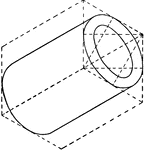Hollow Cylinder

Illustration of the isometric of a hollow cylinder.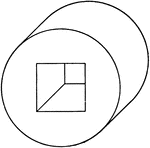Oblique View Of Hollow Cylinder

Illustration of an oblique view of a hollow cylinder. The portion removed from the center of the cylinder…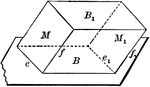Equal and Parallel Opposite Faces of a Parallelopiped

Diagram used to prove the theorem: "The opposite faces of a parallelopiped are equal and parallel."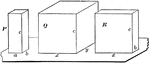Relationship Between 2 Parallelopipeds With Equal Altitudes

Diagram used to prove the theorem: "The rectangular parallelopipeds which have two dimensions in common…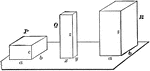Relationship Between Dimensions of Parallelopipeds

Diagram used to prove the theorem: "The rectangular parallelopipeds are to each other as the product…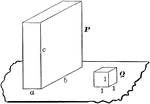Volume of Rectangular Parallelopiped

Diagram used to prove the theorem: "The volume of a rectangular parallelopiped is equal to the product…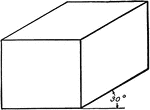Oblique View Of Rectangular Solid

Illustration of an oblique view of a rectangular solid/prism at 30°.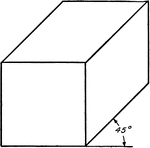Oblique View Of Rectangular Solid

Illustration of an oblique view of a rectangular solid/prism at 45°.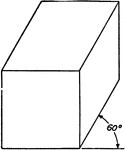Oblique View Of Rectangular Solid

Illustration of an oblique view of a rectangular solid/prism at 60°.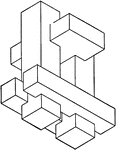Combination of Geometric Solids

Illustration of model created by combining non regular geometric solids.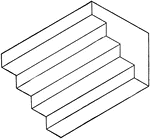Isometric of Stairs

Illustration of stairs; 3-dimensional view of 4 steps. The staircase can be created by combining/stacking…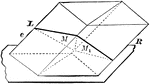Parallelopiped Divided Into Triangular Prisms

Diagram used to prove the theorem: "The plane passed through two diagonally opposite edges of a parallelopiped…